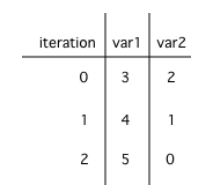Time estimate: 45 min.

# 4.5. Loop Analysis¶

In this lesson, you will practice tracing through code with loops and analyzing loops to determine how many times they run.

## 4.5.1. Tracing Loops¶

Let’s practice tracing through loops with many variables. Remember to make a tracing table to keep track of all the variables, the iterations, and the output.

Here is a complex loop. See if you can trace the code on paper by making a tracing table to predict what the code will do when you run it. Click on the this Java visualizer link or the Code Lens button to help you step through the code.

Can you trace through this code? Add in output statements System.out.println("var1: " + var1 + " var2: " + var2); before the loop and inside the loop at the end to keep track of the variables and run. Click on the Code Lens button to visualize the code step by step.

Did your trace table look like the following?Figure 1: A table showing the values of all of the variables each time through the loop. The 0 means before the first loop.¶Check your understanding

## 4.5.2. Counting Loop Iterations¶

Loops can be also analyzed to determine how many times they run. This is called run-time analysis or a statement execution count.

How many stars are printed out in this loop? How many times does the loop run? Figure it out on paper before you run the code.

If you made a trace table, you would know that the loop runs when i = 3, 4, 5, 6 but finishes as soon as i becomes 7 since that is not less than 7. So, the loop runs 4 times. Or you can use the shortcut formula in the note below.

Note

The number of times a loop executes can be calculated by (largestValue - smallestValue + 1).

• If the loop uses counter <= limit, limit is the largest value.

• If the loop uses counter < limit, limit-1 is the largest value that allows the loop to run.

In the code above the largest value that allows the loop to run is 6 (which is the largest value < 7) and the smallest value that allows the loop to execute is 3 so this loop executes (6 - 3 + 1 = 4 times).

How many stars are printed out by the following loops? How many times do the loops run? Calculate on paper before you run the code.

Note

The number of times a nested for loop body is executed is the number of times the outer loop runs multiplied by the number of times the inner loop runs (outer loop runs * inner loop runs).

For the example above, the outer loop executes 4 - 0 + 1 = 5 times and the inner 9 - 0 + 1 = 10 times so the total is 5 * 10 = 50.

## 4.5.3.Programming Challenge : POGIL Analyzing Loops¶

We encourage you to do this activity as a POGIL (Process Oriented Guided Inquiry Learning) group activity. POGIL groups are self-managed teams of up to 4 students where everyone has a POGIL role and works together to solve the problems, making sure that everyone in the team participates and learns.

Do the following exercises in your group. Make sure you draw the trace tables keeping track of all the variables in the loops. Use the formulas to determine how many times the loops run. If your group finishes early, do some of the multiple-choice problems in the 4.6 Practice and Summary section of this unit.

## 4.5.4. Summary¶

• A trace table can be used to keep track of the variables and their values throughout each iteration of the loop.

• We can determine the number of times a code segment will execute with a statement execution count. This is called run-time analysis.

• The number of times a loop executes can be calculated by largestValue - smallestValue + 1 where these are the largest and smallest values of the loop counter variable possible in the body of the loop.

• The number of times a nested for-loop runs is the number of times the outer loop runs times the number of times the inner loop runs.

## 4.5.5. Loop Analysis Game¶

Try the game below to practice loop analysis. Click on Loops and click on the number of times the loop runs. For an added challenge, try the check boxes for Backwards, Do While, and Nested. We encourage you to work in pairs and see how high a score you can get.